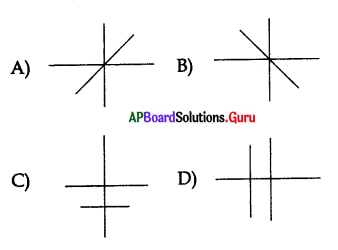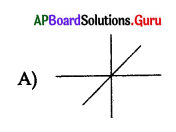Practice the AP 10th Class Maths Bits with Answers Chapter 7 Coordinate Geometry on a regular basis so that you can attempt exams with utmost confidence.

## AP State Syllabus 10th Class Maths Bits 7th Lesson Coordinate Geometry with Answers

Question 1.
If a line is passing through (2, 3) and (2, -3), then
1) the line is parallel to X – axis.
2) the line is parallel to Y – axis.
3) the slope of the line is not defined.
4) the slope of the line is zero.
A) 2 and 3 are correct.
B) 1 and 3 are correct.
C) 1 and 2 are correct.
D) 2 and 4 are correct.
A) 2 and 3 are correct.

Question 2.
If A(log28, log525) and B(log1010, log10100), then the mid-point of AB is
A) (2, 2)
B) (3, 2)
C) (1, 2)
D) (4, 4)
D) (4, 4)Question 3.
The nearest point to origin is
A) (2, -3)
B) (5, 0)
C) (0,-5)
D) (1, 3)
A) (2, -3)

Question 4.
The point (0, 5) lies on ……………….
A) Both X and Y – axes
B) Origin
C) Y-axis
D) X – axis
C) Y-axis

Question 5.
If origin is the centroid of a triangle, whose vertices are (3, 2), (-6, y) and (3,-2), then y = ………………….
A) 0
B) 3
C) 2
D) 6
A) 0

Question 6.
Which of the following cannot be a point on X-axis ?
A) (-2, 0)
B) (0, 2)
C) (2, 0)
D) (4, 0)
B) (0, 2)

Question 7.
Which of the following is the point of intersection of X – axis and the line y = x + 5?
A) (0, 5)
B) (5, 0)
C) (0, -5)
D) (-5, 0)
D) (-5, 0)

Question 8.
The area of the triangle BOA is ……………… sq units.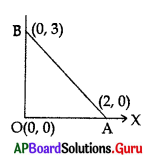A) 1
B) 2
C) 3
D) 4
C) 3

Question 9.
The distance between two points A(a cosθ, 0), B(0, asinθ) is ……………….
A) a
B) a2
C) √a
D) 0
A) aQuestion 10.
(x, y), (2, 0), (3, 2) and (1, 2) are vertices of a parallelogram then (x, y)
A) (0, 0)
B) (4, 8)
C) (1, 0)
D) (5, 0)
A) (0, 0)

Question 11.
Total distance from X-axis to A(3, 4) and Y-axis to B(5, 7) is ……………………..
A) 8
B) 10
C) 11
D) 9
D) 9

Question 12.
Distance between the points A(log10100, tan 45°),
B(cosec 30°.log7343) is
A) $$\sqrt{13}$$T units
B) $$\sqrt{10}$$ units
C) $$\sqrt{34}$$ units
D) √5 units
D) √5 units

Question 13.
The point (-5, -8) lies in ………. quadrant.
A) I
B) II
C) III
D) IV
C) III

Question 14.
The line x = -3 is
A) parallel to X – axis
B) parallel to Y – axis
C) passes through origin
D) passes through (0, -3)
B) parallel to Y – axis

Question 15.
The area of a triangle is zero means …………………
A) points are parallel
B) points are collinear
C) points are equal
D) points are negative
B) points are collinear

Question 16.
The formula of the area of a triangle is ………………….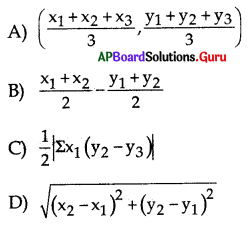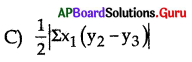Question 17.
Slope of the line 3x – αy +1 = 0 is 4 then α = …………………..
A) $$\frac {3}{4}$$
B) $$\frac{-3}{4}$$
C) $$\frac {1}{3}$$
D) $$\frac{-1}{3}$$
D) $$\frac{-1}{3}$$Question 18.
Sides of two similar triangles are in the ratio of 4:9. Area of these triangles are in the ratio of ………………
A) 2 : 3
B) 4 : 9
C) 81 : 16
D) 16 : 81
D) 16 : 81

Question 19.
The point at which the line 2x + y = 8 intersects the X-axis is ………………….
A) (8, 0)
B) (0, 8)
C) (0, 4)
D) (4, 0)
D) (4, 0)

Question 20.
Three consecutive vertices of a parallelogram are (-2, 1), (1, 0) and (4, 3). Then 4th vertex is ………………
A) (1, 4)
B) (1, -2)
C) (-1, 2)
D) (-1, -2)
A) (1, 4)

Question 21.
The ratio in which the line segment joining the points (4, 6) and (-7, -1) divided by X- axis is ………………….
A) 6 : 1
B) 1 : 6
C) 4 : 7
D) 7 : 4
A) 6 : 1

Question 22.
Slope of the line joining (-α, α) and (α, α + α√3) is …………………
A) $$\frac{2}{\sqrt{3}}$$
B) $$\frac{1}{\sqrt{3}}$$
C) √3
D) $$\frac{\sqrt{3}}{2}$$
D) $$\frac{\sqrt{3}}{2}$$

Question 23.
If the centroid of the triangle formed with (a, b), (b, c) and (c, a) is O(0,0), then
a3 + b3 + c3 =
A) a + b + c
B) $$\frac{a+b+c}{3}$$
C) $$\frac{a b c}{3}$$
D) 3abc
D) 3abc

Question 24.
If A(p, q), B(m, n) and C(p-m, q-n) are collinear, then……………….
A) $$\frac{\mathrm{p}}{\mathrm{n}}=\frac{\mathrm{q}}{\mathrm{m}}$$
B) $$\frac{\mathrm{p}}{\mathrm{m}}=\frac{\mathrm{q}}{\mathrm{n}}$$
C) p + q = m + n
D) p + q + m + n = 0
B) $$\frac{\mathrm{p}}{\mathrm{m}}=\frac{\mathrm{q}}{\mathrm{n}}$$

Question 25.
If P = {3x/x ∈N,x ≤ 50},
Q = {5x/x ∈N, x ≤ 30}, then n(P ∩ Q) =……………..
A) 5
B) 8
C) 10
D) 15
C) 10Question 26.
(3, -1), (2, 6) and (-5, 7) are the midpoints of the sides of the triangle ABC. The area of triangle ABC is ………………. sq. units
A) 96
B) 69
C) 86
D) 110
A) 96

Question 27.
The ratio in which X-axis divides the line segment joining the points (5, 4) and (5, -3) is ……………..
A) 5 : 2
B) 3 : 4
C) 2 : 5
D) 4 : 3
D) 4 : 3

Question 28.
AOBC is a rectangle with A = (0, 3), O (0, 0) and B (5, 0), then the length of
its diagonals is ………………
A) 5
B) 3
C) $$\sqrt{34}$$
D) 4
C) $$\sqrt{34}$$

Question 29.
The points (-4, 0), (4, 0), (0, 3) are vertices of ……………….
A) right angled triangle
B) isosceles triangle
C) equilateral triangle
D) scalene triangle
B) isosceles triangle

Question 30.
In the given figure AC pass through center of the circle, then the area of the shaded region
region in the given figure is ………………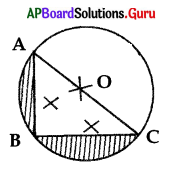A) $$\frac{x^{2}}{2}$$ (3 – π)
B) x2($$\frac{\pi}{2}$$ – 1)
C) 2x2(π – 1 )
D) $$\frac{x^{2}}{2}\left(\frac{\pi}{2}-1\right)$$
D) $$\frac{x^{2}}{2}\left(\frac{\pi}{2}-1\right)$$

Question 31.
The points of trisection of the line segment joining (2, -2), (-7, 4) are
A) (1, 0), (-4, 2)
B) (-1, 0), (-4, 2)
C) (-1, 0), (-4, -2)
D) (1, 0), (4, 2)
B) (-1, 0), (-4, 2)

Question 32.
The points which divide the line segment joining A(-2, 2) and B(2, 8) into four equal parts are)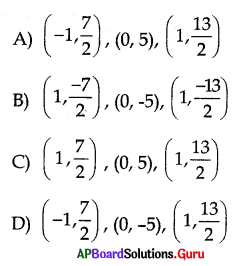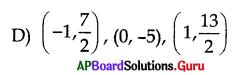Question 33.
The end points of a line are (2, 3), (4, 5). Then its slope is
A) 4
B) 3
C) 2
D) 1
D) 1Question 34.
The value of k for which the points (7, -2), (5, 1), (3, k) are collinear is
A) 4
B) 3
C) 2
D) 1
A) 4

Question 35.
The points A(7, 3), B(6, 1), C(8, 2) and D(9, 4) taken in that order are the vertices of
A) square
B) rhombus
C) parallelogram
D) trapezium
B) rhombus

Question 36.
If (a, 2) lies in II quadrant, then (-a, -2) lies in which quadrant ?
A) I
B) II
C) III
D) IV
D) IV

Question 37.
The quadrilateral formed by the points A(0, -1), B(2, 1), C(0, 3) and D(-2, 1) taken in the same order is
A) rectangle
B) parallelogram
C) square
D) rhombus
C) square

Question 38.
If P(3, 4) and Q(7, 7) are two points and PR = 10, where P, Q and R are collinear, then R =
A) (10,10)
B) (11, 11)
C) (11, 10)
D) (11, -10)
C) (11, 10)

Question 39.
If (-2, 1), (1, 0) and (4, 3) are three consecutive vertices of a parallelogram, then the fourth vertex is
A) (2, 1)
B) (1, 4)
C) (0, 0)
D) (2, 2)
A) (2, 1)

Question 40.
(0, 0), (1, 0), (0, -4) are the vertices of a …………….. triangle.
A) equilateral
B) isosceles
C) right-angled isosceles
D) right-angled
D) right-angledQuestion 41.
If (8,1), (k, -4), (2, -5) are collinear, then k =
A) 1
B) 2
C) 3
D) 4
C) 3

Question 42.
If the slope of the line through (2, -7) and (x, 5) is 3 then x =
A) 4
B) 5
C) 6
D) 7
C) 6

Question 43.
The distance between the points (cosθ, sinθ) and (-sinθ, cosθ) is
A) 1
B) √2
C) 2
D) √3
B) √2

Question 44.
The number of elements in a triangle is
A) 3
B) 4
C) 6
D) 5
C) 6

Question 45.
The graph of y + x2 = 0 lies in the quadrants
A) Q1, Q2
B) Q2, Q3
C) Q3, Q4
D) Q4, Q1
C) Q3, Q4

Question 46.
The line x = 2017 is
A) Slope not defined
B) Parallel to Y – axis
C) 1 and 2
D) None
C) 1 and 2

Question 47.
If (k, 2) lies in II quadrant then (-k, -2) lies in the quadrant
A) I
B) II
C) III
D) IV
D) IVQuestion 48.
The points A(1, 7), B(4, 2), C(-1, -1) and D(-4, 4) are
A) collinear
B) vertices of a square
C) vertices of a rectangle
D) None of these
B) vertices of a square

Question 49.
If P divides the line joining A(x1, y1) and B(x2, y2) internally in the ratio m1 : m2, then P is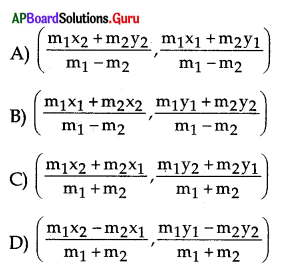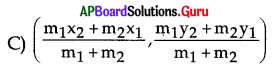Question 50.
The distance between the points A(4, 3) and B(8, 6) is …………… units.
A) 5
B) 6
C) 4
D) 7
C) 4

Question 51.
The points A(1, 5), B(2, 3) and C(-2, -1) are
A) vertices of a right-angled triangle
B) vertices of an isosceles triangle
C) collinear
D) non-collinear
D) non-collinear

Question 52.
The midpoint of A(1, 5) and B (-2, -1) is
A) ($$\frac {1}{2}$$, 2)
B) (2, $$\frac {1}{2}$$)
C) (-$$\frac {1}{2}$$, 2)
D) (-$$\frac {1}{2}$$, -2
C) (-$$\frac {1}{2}$$, 2)

Question 53.
If a line makes an angle 45° with positive X-axis, then its slope is
A) $$\frac{1}{\sqrt{2}}$$
B) $$\frac {1}{2}$$
C) $$\frac{\sqrt{3}}{2}$$
D) 1
A) $$\frac{1}{\sqrt{2}}$$

Question 54.
The area of the triangle formed by three vertices O(0, 0), A(1, 0), B(0, 1) is ………………. sq. units.
A) o
B) 1/2
C) 1
D) 2
B) 1/2

Question 55.
At which point does the straight line y = x + 7 intersect X – axis ?
A) (7, 0)
B) (0, 7)
C) (0, -7)
D) (-7, 0)
D) (-7, 0)Question 56.
Where do the following points (-4, 0), (2, 0), (6, 0), (-8, 0) lie in the coordinate axes ?
B) Y-axis
C) X-axis
C) X-axis

Question 57.
The distance between the points (0, 0), (X1,Y1) is ………………units.
A) $$\sqrt{x_{1}^{2}+y_{1}^{2}}$$
B) $$\sqrt{x_{1}+y_{1}}$$
C) $$\sqrt{x^{2}+y^{2}}$$
D) $$\sqrt{x+y}$$
A) $$\sqrt{x_{1}^{2}+y_{1}^{2}}$$

Question 58.
The slope of a ladder making an angle 60° with the floor is ……………………..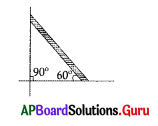A) 1
B) $$\frac {1}{2}$$
C) $$\frac{1}{\sqrt{3}}$$
D) √3
D) √3

Question 59.
The mid point of the line joining the points (4, 5), (- 6, 3) is ………………
A) (1, 4)
B) (-1, 4)
C) (1, -4)
D) (-1, – 4)
B) (-1, 4)

Question 60.
The distance of the point (3, – 4) from X- axis is ………………..
A) 3
B) 4
C) 5
D) 1
C) 5

Question 61.
The slope of the line joining (2, 1) (3, 6) is …………………..
A) 5
B) 4
C) 3
D) 2
A) 5Question 62.
The line passing through the point (0,0) is
A) y = 2
B) x = 5
C) y = x
D) y = 2x + 3
C) y = x

Question 63.
Slope of the line 5x – 6y + 10 = 0 is
A) $$\frac{5}{6}$$
B) $$\frac{-5}{6}$$
C) $$\frac{6}{5}$$
D) $$\frac{-6}{5}$$
A) $$\frac{5}{6}$$

Question 64.
The distance between the points (3, 2) and (2, 4) is …………………. units.
A) √5
B) √6
C) √3
D) √2
A) √5

Question 65.
The centroid of the triangle with vertices (2, 1), (3, 2), (1, 3) is
A) (3, 5)
B) (2, 2)
C) (3, 3)
D) (3, 6)
B) (2, 2)

Question 66.
If the line 4x + 5y = k passing through the origin, then k is
A) -1
B) 2
C) 0
D) 3
C) 0

Question 67.
The area of the triangle whose vertices are (0, 0), (x, 0) and (0, y) is
A) xy
B) $$\frac {1}{2}$$ xy
C) $$\frac {1}{2}$$ x + y
D) x + y
B) $$\frac {1}{2}$$ xy

Question 68.
The slope of the line perpendicular to the line 2x + y + 4 = 0 is
A) -2
B) 2
C) $$\frac {1}{2}$$
D) –$$\frac {1}{2}$$
D) –$$\frac {1}{2}$$Question 69.
The slope of the line parallel to the line 2x + y + 4 = 0 is
A) 2
B) -2
C) $$\frac {1}{2}$$
D) –$$\frac {1}{2}$$
A) 2

Question 70.
The equation of the line having slope 1 and passing through (3, 2) is
A) x + y = 1
B) x – y = 1
C) 2x – y + 1 = 0
D) x – 2y + 1 = 0
B) x – y = 1

Question 71.
Slope of the line joining the points (a, 0) and (0, 5) is
A) -b
B) $$\frac{a}{b}$$
C) – $$-\frac{b}{a}$$
D) $$\frac{1}{a}$$
C) – $$-\frac{b}{a}$$

Question 72.
The distance of the point (3, p) from origin is 5 units then p is
A) 7
B) 6
C) 5
D) 4
D) 4

Question 73.
A = (8, 3), B= (x, 3) and $$\overline{\mathbf{A B}}$$ = 12 units then x is
A) -4
B) 14
C) 8
D) 20
D) 20

Question 74.
Distance of the point (tanθ, 1) from origin is
A) cotθ
B) sinθ
C) tanθ
D) secθ
D) secθ

Question 75.
The perimeter of a triangle formed by the points (0,0), (1,0)and (0, 1) is ………………….units.
A) √2
B) 2 + √2
C) 2 – √2
D) 2 √2
B) 2 + √2Question 76.
Find the value of k for which of (7, -2), (5, 1), (3, k) are collinear
A) $$\frac {4}{5}$$
B) $$\frac {11}{5}$$
C) $$\frac {5}{5}$$
D) $$\frac {7}{5}$$
B) $$\frac {11}{5}$$

Question 77.
Find the centroid of (-1, 3), (6, -3), (-3, 6)
A) ($$\frac {2}{3}$$, 2)
B) (1, $$\frac {2}{3}$$)
C) (-1, $$\frac {2}{3}$$)
D) (-$$\frac {2}{3}$$, 1)
A) ($$\frac {2}{3}$$, 2)

Question 78.
A = (4, 2); B = (1, y) and AB = 5 then y = ………………..
A) 6
B) 3
C) 9
D) 2
A) 6

Question 79.
A straight line which is parallel to x – y + 1 = 0 is ………………..
A) x – y + k = 0
B) x + y + k = 0
C) ax + by + c = 0
D) 2x + 3y + k = 0
A) x – y + k = 0

Question 80.
If the distance of a point from X – axis is 8 units and its distance from Y – axis is 3 units then the point is denoted by
A) (8, 3)
B) (-8, 3)
C) (-3, 8)
D) (3, 8)
D) (3, 8)

Question 81.
The slope of the line parallel to 3x – by + 7 = 0 is 3 then b = ………………..
A) 3
B) 4
C) 1
D) 5
C) 1

Question 82.
The slope of the line perpendicular to 13x – 7y + 1 = 0 is ……………….
A) $$\frac{-7}{13}$$
B) $$\frac{7}{3}$$
C) –$$\frac{7}{3}$$
D) $$\frac{13}{7}$$
A) $$\frac{-7}{13}$$

Question 83.
The coordinates of the centre of the circle having the points (9, 3) and (1, -1) as the end points of the diameter is ………………..
A) (6, 9)
B) (5, 1)
C) (1, 3)
D) (- 1, – 3)
B) (5, 1)Question 84.
One end of the diameter of a circle is (3, 2) and the centre is (0, 0) then the other end point is
A) (8, 4)
B) (3, 2)
C) (- 3, – 2)
D) (5, 1)
C) (- 3, – 2)

Question 85.
The mid point of the line segment (3, x) and (1, – 4) is (2, 1) then x =
A) 2
B) – 1
C) 6
D) 0
C) 6

Question 86.
The point that lies in IV quadrant
A) (2, 3)
B) (-3, -2)
C) (4, -3)
D) (-2, 3)
C) (4, -3)

Question 87.
The point that lies in III quadrant
A) (5, 4)
B) (-5, -4)
C) (-5, 4)
D) (5, -4)
B) (-5, -4)

Question 88.
The point that lies in II quadrant is ………………
A) (-2, 3)
B) (2, -3)
C) (2, 3)
D) (0, 0)
A) (-2, 3)

Question 89.
x > 0, y > 0, then (x, y) ∈ ………………..
A) Q1
B) Q2
C) Q3
D) Q4
A) Q1

Question 90.
x < 0, y > 0 then (x, y) ∈ ……………..
A) Q1
B) Q2
C) Q3
D) Q4
B) Q2Question 91.
x < 0, y < 0 then (x, y) ∈ ………………
A) Q1
B) Q2
C) Q3
D) Q4
C) Q3

Question 92.
x > 0, y < 0 then (x, y) ∈ ……………..
A) Q1
B) Q2
C) Q3
D) Q4
D) Q4

Question 93.
Slope of the line $$\frac{x}{a}+\frac{y}{b}$$ = 1 is
A) $$\frac{1}{b}$$
B) $$\frac{1}{a}$$
C) $$\frac{a}{b}$$
D) –$$\frac{\mathrm{b}}{\mathrm{a}}$$
D) –$$\frac{\mathrm{b}}{\mathrm{a}}$$

Question 94.
Slope of the line x = 9 is
A) 9
B) – 9
C) 0
D) 5
A) 9

Question 95.
The distance between the pointsP(x, y) from origin O (0, 0) is
A) $$\sqrt{x+y}$$
B) $$\sqrt{x^{2}+y^{2}}$$
C) x2 + y2
D) x + y
B) $$\sqrt{x^{2}+y^{2}}$$

Question 96.
A point on the X – axis
A) (3, 3)
B) (4, 5)
C) (2, 0)
D) (0, 2)
C) (2, 0)

Question 97.
A point on the Y – axis
A) (4, 5)
B) (0, 3)
C) (3, 0)
D) (4, 3)
B) (0, 3)Question 98.
The ratio in which the centroid divides the median of a triangle is
A) 1 : 2
B) 2 :1
C) 1 : 3
D) 3 : 1
C) 1 : 3

Question 99.
Intercept form of a line is …………….
A) $$\frac{x}{a}+\frac{y}{b}$$ = 1
B) x – y = 7
C) $$\frac{x}{a}+\frac{y}{b}$$ = 1
D) y = mx + c
A) $$\frac{x}{a}+\frac{y}{b}$$ = 1

Question 100.
X – intercept of the line ax + by + c = 0 is
A) $$\frac{-\mathrm{c}}{\mathrm{a}}$$
B) $$\frac{c}{a}$$
C) $$\frac{a}{c}$$
D) $$\frac{1}{c}$$
C) $$\frac{a}{c}$$

Question 101.
The line y = mx + c meets Y – axis at the point ……………..
A) (0, 0)
B) (c, 0)
C) (0, c)
D) (0, m)
C) (0, c)

Question 102.
The line y = mx + c meets X – axis at the point ………………..
A) (0, 0)
B) (c, 0)
C) (0, c)
D) (0, m)
B) (c, 0)

Question 103.
Slope of a line can be taken as ………………
A) cosθ
B) tanθ
C) cosecθ
D) sinθ
B) tanθ

Question 104.
The mid point of a line segment divides in the ratio
A) 2 : 1
B) 1 : 2
C) 1 : 1
D) 8 : 7
C) 1 : 1

Question 105.
x = 0 represents …………………
A) X – axis
B) Y – axis
C) (0, 0)
D) y = mx + C
B) Y – axisQuestion 106.
y = 0 represents ………………….
A) X – axis
B) Y – axis
C) (0, 0)
D) y = mx + C
A) X – axis

Question 107.
x = k represents ……………….
A) parallel to X – axis
B) parallel to Y – axis
C) parallel to X, Y axes
D) (0, 0)
B) parallel to Y – axis

Question 108.
y = k represents ………………
A) parallel to X – axis
B) parallel to Y – axis
C) parallel to X, Y axes
D) (0, 0)
A) parallel to X – axis

Question 109.
In the coordinate plane the axes are ……………. to each other.
A) parallel
B) perpendicular
C) either perpendicular or parallel
D) neither parallel nor perpendicular
B) perpendicular

Question 110.
The distance of a point from Y – axis is called …………………..
A) y – coordinate
B) x – coordinate
C) origin
D) (0, 0)
B) x – coordinate

Question 111.
The distance of a point from X – axis is called ………………..
A) x – coordinate
B) y – coordinate
C) origin
D) coordinate
B) y – coordinate

Question 112.
The coordinates of origin are
A) (0, 0)
B) (1, 1)
C) (1, 0)
D) (0, 1)
A) (0, 0)Question 113.
The point which is not on the X – axis
A) (-1, 0)
B) (8, 0)
C) (0, 8)
D)(-2, 0)
C) (0, 8)

Question 114.
The point which is not on the Y – axis is
A) (-2, 0)
B) (0, 2)
C) (0, -3)
D) (0, 4)
A) (-2, 0)

Question 115.
Area of a triangle enclosed by the axis and the line $$\frac{x}{a}+\frac{y}{b}$$ = 1 is a b
A) $$\frac{\mathrm{ab}}{3}$$
B) $$\frac{a^{2} b^{2}}{2}$$
C) $$\frac{\mathrm{ab}}{2}$$
D) $$\frac{a+b}{2}$$
C) $$\frac{\mathrm{ab}}{2}$$

Question 116.
If G is the centroid of ΔABC, then ΔGAB = ……………….
A) $$\frac {1}{13}$$ABC
B) $$\frac {1}{4}$$ABC
C) $$\frac {1}{2}$$ABC
D) $$\frac {1}{3}$$ABC
D) $$\frac {1}{3}$$ABC

Question 117.
The two lines x = 2 and y = -3 will meet at ………………….
A) (1, 8)
B) (2, 4)
C) (-2, 1)
D) (2, -3)
D) (2, -3)

Question 118.
Angle made by the line y = x with the positive direction of X – axis is
A) 80°
B) 45°
C) 90°
D) 100°
B) 45°

Question 119.
To locate the exact position of a point the number of references we need is ……………….
A) 0
B) 1
C) 2
D) 3
C) 2Question 120.
In a coordinate system the horizontal line is called ………………………
A) Y – axis
B) X – axis
C) origin
D) reference
B) X – axis

Question 121.
In a coordinate system the vertical line is called …………………..
A) Y – axis
B) X – axis
C) origin
D) reference
A) Y – axis

Question 122.
The meeting point of the two axis in the coordinate plane is called
A) origin
B) X – axis
C) Y – axis
D) (2, 3)
A) origin

Question 123.
The point which lies on both the axis is
A) (1, 1)
B) (2, 2)
q (-1, -1)
D) (0, 0)
D) (0, 0)

Question 124.
The x – coordinate of a point is also called
A) ordinate
B) 2nd coordinate
C) abscissa
D) origin
C) abscissa

Question 125.
The y – coordinate of a point is also called
A) ordinate
B) abscissa
C) 1st coordinate
D) origin
A) ordinate

Question 126.
The coordinates of a point are (0,0) then the point is
A) origin
B) X – axis
C) ordinate
D) abscissa
A) origin

Question 127.
Indentify the point in Q1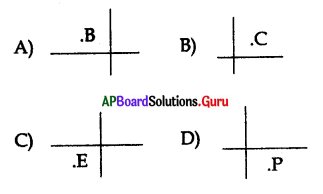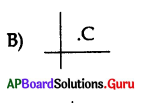Question 128.
A line parallel to x = 3 is ………………….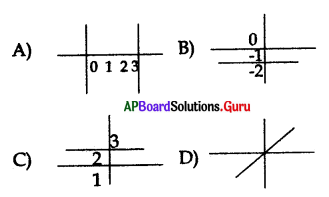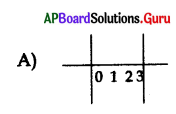Question 129.
Which of the following line passing through (0,0) ?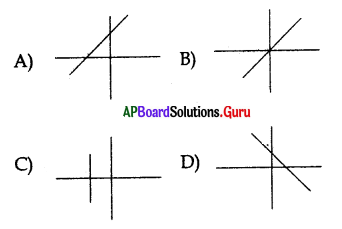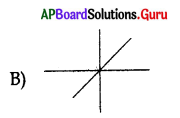Question 130.
The location of points (1, 1)(2, 2)(3, 3)in a graph sheet may represents as ………………..